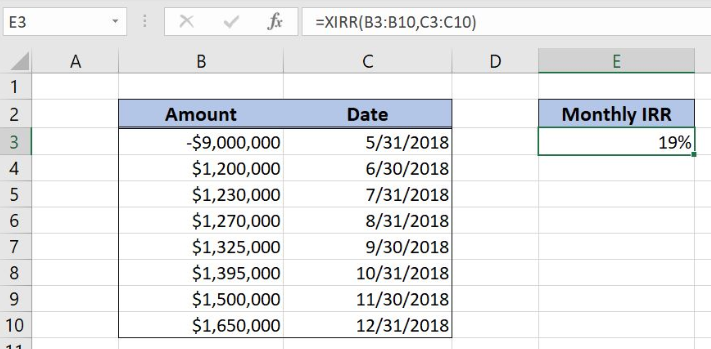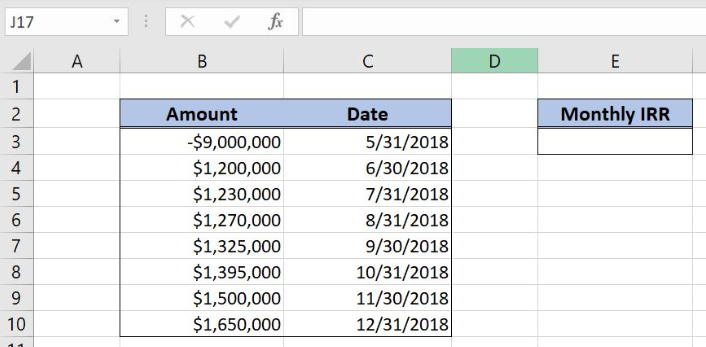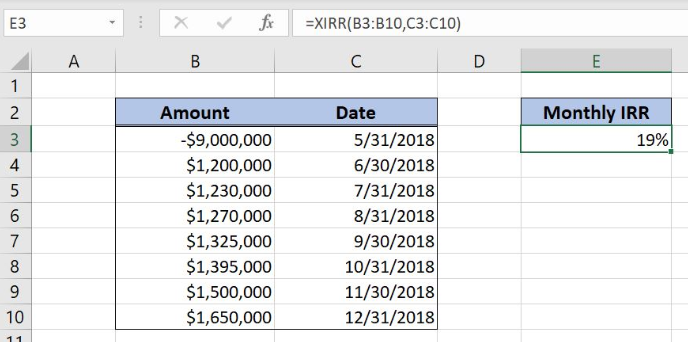Get instant live expert help with Excel or Google Sheets“My Excelchat expert helped me in less than 20 minutes, saving me what would have been 5 hours of work!”

Post your problem and you’ll get expert help in seconds.

Your message must be at least 40 characters
Our professional experts are available now. Your privacy is guaranteed.
All articles IRR Learn How to Calculate the Monthly IRR in Excel

Learn How to Calculate the Monthly IRR in Excel

Excel allows a user to get the monthly internal rate of return of an investment using the XIRR function. With defined monthly periods, we will get the exact IRR. This step by step tutorial will assist all levels of Excel users to learn how to calculate the monthly IRR in Excel.Figure 1. The result of the XIRR function

Syntax of the XIRR Formula

The generic formula for the XIRR function is:

`=XIRR (values, dates, [guess])`

The parameter of the XIRR function is:

• values – a range of cells containing values, including initial investment and incomes. The investment must have a negative sign, as it is a cost
• dates – a range of dates corresponding to values. All dates must be in chronological order. In our case, we put the last day of every month
• [guess] – an estimated value for the expected XIRR. This parameter is non-mandatory. If it’s omitted, the function will take a default value of 0.1 (=10%).

Setting up Our Data for the XIRR Function

Let’s look at the structure of the data we will use. In column B (“Amount”), we have values including initial investment and periodical incomes. In column C (“Date”) we have a corresponding date for every amount. In the cell E3, we want to get the XIRR.Figure 2. Data that we will use in the example

Get the Monthly IRR Using the XIRR Function

In our example, we want to get the XIRR of the values in the range B3:B10. The result will be in the cell E3.

The formula looks like:

`=XIRR(B3:B10, C3:C10)`

The parameter values is the range B3:B10 and the parameter dates is C3:C10.

To apply the XIRR function, we need to follow these steps:

• Select cell E3 and click on it
• Insert the formula: `=XIRR(B3:B10, C3:C10)`
• Press enter.Figure 3. Using the XIRR function to get the internal rate of the investment

Finally, the result in the cell E3 is 19%, which is the monthly internal rate of the investment.

Most of the time, the problem you will need to solve will be more complex than a simple application of a formula or function. If you want to save hours of research and frustration, try our live Excelchat service! Our Excel Experts are available 24/7 to answer any Excel question you may have. We guarantee a connection within 30 seconds and a customized solution within 20 minutes.Subscribe to Excelchat.co